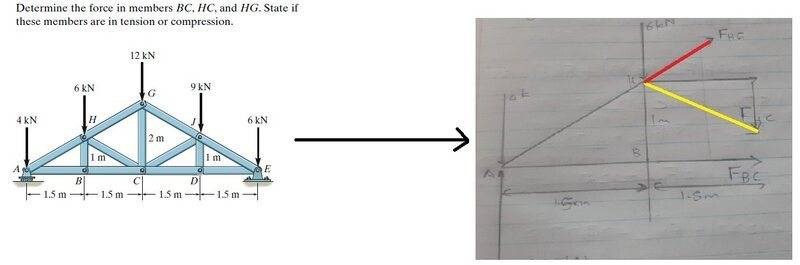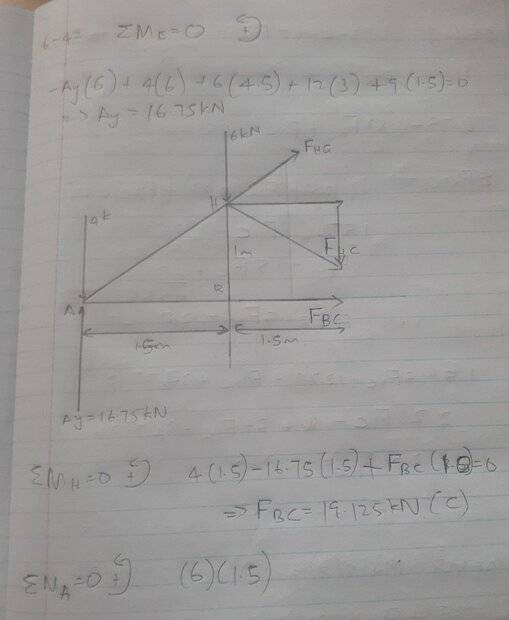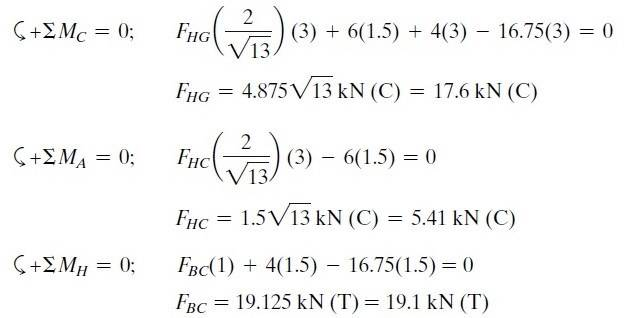# Using method of sections to solve for truss systems

• Engineering
• user12323567
In summary, the conversation discusses the use of a 3 meter distance in calculating moments about points A and C in relation to Fhc and Fhg. The perpendicular distance between the extended line of action of Fhc and the pivot A is 1.66 m, and the line of action is at an angle of 33.69° with respect to AC. The summation of moments about A shows the equivalent to 1.66 multiplied by Fhc, as well as the load of 6 KN properly multiplying its perpendicular distance to A of 1.5 m. The expert also explains the use of equivalent triangles and the alignment of internal forces with the pivot points. Finally, the expert provides additional resources for further understanding off

#### user12323567

Homework Statement
Determine the force in members BC, HC, and HG. State if these members are in tension or compression.
Relevant Equations
Rigid-body assumption
Taking the moments about points A and C, why is a distance of 3 meters used in Fhc and Fhg? Isn’t it supposed to be 1.5 meters for both?
I acknowledge that Fhc and Fhg have x and y components, but were we considering the horizontal or vertical component (or both components) of either Fhc and Fhg in taking moments about points A and C respectively?The perpendicular distance between the extended line of action of Fhc and the pivot A is 1.66 m.
That line of action is at an angle of 33.69° respect to AC.
The summation of moments about A in the provided response shows the equivalent to 1.66 multiplied by Fhc, as well as the load of 6 KN properly multiplying its perpendicular distance to A of 1.5 m.

The perpendicular distance between the extended line of action of Fhc and the pivot A is 1.66 m.
That line of action is at an angle of 33.69° respect to AC.
The summation of moments about A in the provided response shows the equivalent to 1.66 multiplied by Fhc, as well as the load of 6 KN properly multiplying its perpendicular distance to A of 1.5 m.
Yes, you take 2/sqrt(13) and multiply it by 3 to get said 1.66 m, I am asking why you are multiplying by 3 instead of 1 (the vertical perpendicular distance between A and Fhc)

Yes, you take 2/sqrt(13) and multiply it by 3 to get said 1.66 m, I am asking why you are multiplying by 3 instead of 1 (the vertical perpendicular distance between A and Fhc)
Since Fhc is at an angle, do we consider both its components when we are looking at the moment about point A? i.e. Fhc(1.5)sin(gamma) - Fhc(1)cos(gamma)? Gamma is the angle of Fhc

How is that vertical distance perpendicular to Fhc?
Please, take a step back and see how the forces aligned with the links try to rotate the armature about pivot A.
There is no need to break any of those forces into its x and y components.

You have two equivalent triangles to work with: ACH and ABH.
Because each node in the structure is a pivot, those internal forces of the links can only exist in alignment with them.

Last edited:
How is that vertical distance perpendicular to Fhc?
Please, take a step back and see how the forces aligned with the links try to rotate the armature about pivot A.
There is no need to break any of those forces into its x and y components.

You have two equivalent triangles to work with: ACH and ABH.
Because each node in the structure is a pivot, those internal forces of the links can only exist in alignment with them.
If you consider the horizontal component of Fhc, you have a vertical perpendicular distance of 1. This would be much easier for me to understand if you explained why we have to use a distance of 3 meters when Fhc is not 3 metres away from A, please explain this.

Yes, give me some time, please, and I will get back to you.
Note that, in that case, you also have to consider the additional moment that the vertical component of Fhc induces on A.
Then, the problem asks for the actual Fhc; therefore, you still have to use trigonometry to calculate that value.